### Fast Stylometry Tutorial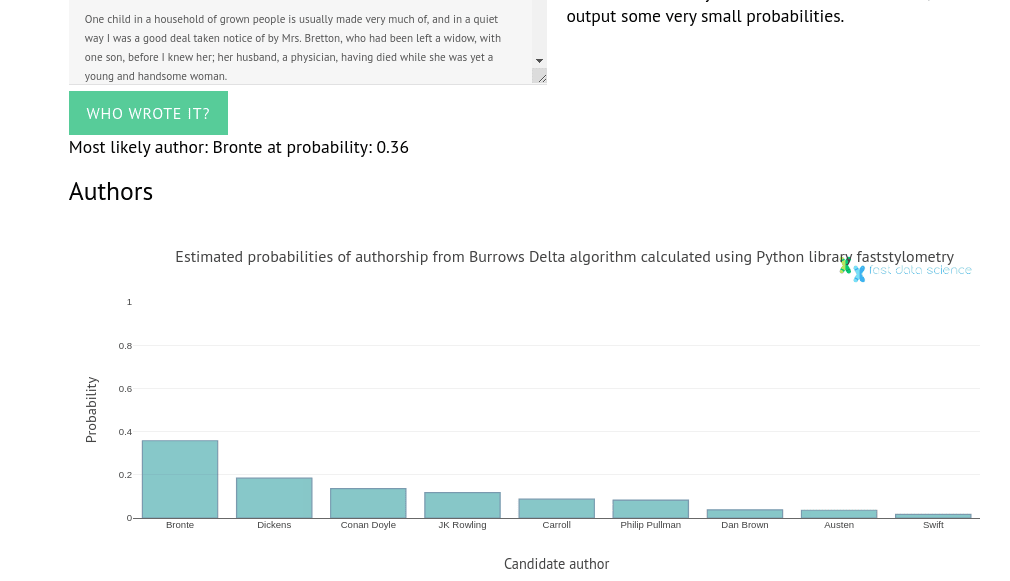Click to try the live demo of the Forensic Stylometry library faststylometry.

I’m introducing a Python library I’ve written, called faststylometry, which allows you to compare authors of texts by their writing style. The science of comparing authors’ fingerprints in this way is called forensic stylometry.

You will need a basic knowledge of Python to run this tutorial.

The faststylometry library uses the Burrows’ Delta algorithm, a well-known stylometric technique. The library can also calculate the probability that two books were by the same author.

I wrote this library to improve my understanding, and also because the existing libraries I could find were focused around generating graphs but did not go as far as calculating probabilities.

Here I am giving a walkthrough of how to conduct a basic stylometry analysis using the faststylometry library. We will test the Burrows’ Delta code on two “unknown” texts: Sense and Sensibility by Jane Austen, and Villette by Charlotte Brontë. Both authors are in our training corpus.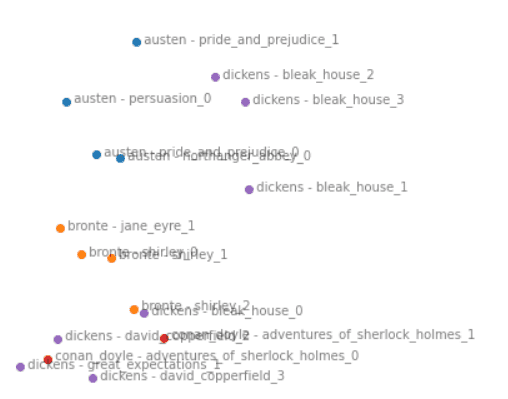Graph showing the proximities of authors’ writing styles calculated using Principal Component Analysis (PCA).

## Burrows’ Delta algorithm

The Burrows’ delta is a statistic which expresses the distance between two authors’ writing styles. A high number like 3 implies that the two authors are very dissimilar, whereas a low number like 0.2 would imply that two books are very likely to be by the same author. Here is a link to a useful explanation of the maths and thinking behind Burrows’ Delta and how it works.

The Burrows’ delta is calculated by comparing the relative frequencies of function words such as “inside”, “and”, etc, in the two texts, taking into account their natural variation between authors.

## Installing faststylometry

If you’re using Python, you can install the library with the following command:

`pip install faststylometry`

The Jupyter notebook for this walkthrough is here.

## Loading the library

First, we start up Python. First we need to import the stylometry library:

```from faststylometry import Corpus
from faststylometry import load_corpus_from_folder
from faststylometry import tokenise_remove_pronouns_en
from faststylometry import calculate_burrows_delta
from faststylometry import predict_proba, calibrate```

The library depends on NLTK (the Natural Language Toolkit), so the first time that you are using it, you may need to run the following commands in your Python environment if you want to use the inbuilt tokeniser:

```import nltk
nltk.download("punkt")```

## Loading some books into a corpus

I have provided some test data for you to play with the library, which you can download from the project Github here. It’s a selection of classic literature from Project Gutenberg, such as Jane Austen and Charles Dickens. Due to copyright, I cannot provide more modern books, but you can always obtain them elsewhere.

If you are using Git, you can download the sample texts with this command:

`git clone https://github.com/fastdatascience/faststylometry`

Make sure the book texts are in the folder faststylometry/data/train on your computer, and each file is named “author name”_-_”book title”.txt, for example: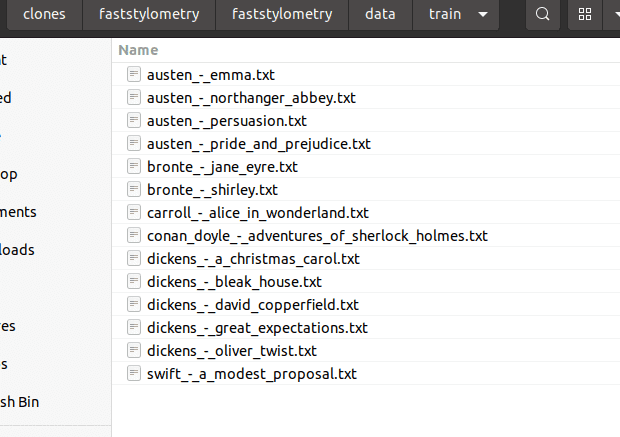The training data supplied in the repository, a selection of English literature from Project Gutenberg, with structured filenames.

You can now load the books into the library, and tokenise them using English rules:

```train_corpus = load_corpus_from_folder("faststylometry/data/train")

train_corpus.tokenise(tokenise_remove_pronouns_en)```

Alternatively, you can add books to your corpus using the following process:

`corpus = Corpus()corpus.add_book("Jane Austen", "Pride and Prejudice", [whole book text])`

## Finding the authorship of an unknown book

I have also provided some “unknown” books for us to test the performance of the algorithm. Imagine we have come across a work for which the author is unknown. The books I’ve included are Sense and Sensibility, written by Jane Austen (but marked as “janedoe”), and Villette, written by Charlotte Brontë, which I have marked as “currerbell”, Brontë’s real pseudonym. They are in the folder faststylometry/data/test.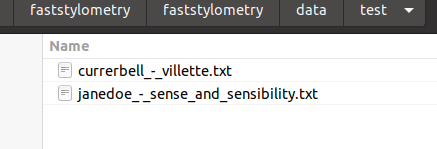The two test documents which I have included in the library. “Currer Bell” is really Charlotte Brontë and “Jane Doe” is really Jane Austen.

Here is the code to load the unknown documents into a new corpus, and tokenise it so that it is ready for analysis:

```# Load Sense and Sensibility, written by Jane Austen (marked as "janedoe")
# and Villette, written by Charlotte Brontë (marked as "currerbell", Brontë's real pseudonym)

test_corpus = load_corpus_from_folder("faststylometry/data/test", pattern=None)
# You can set pattern to a string value to just load a subset of the corpus.

test_corpus.tokenise(tokenise_remove_pronouns_en)
```

## Calculate Burrows’ Delta for both unknown texts

Now we have a training corpus consisting of known authors, and a test corpus containing two “unknown” authors. The library will give us the Burrows’ Delta statistic as a matrix (Pandas dataframe) for both unknown texts (x-axis) vs all known authors (y-axis):

`calculate_burrows_delta(train_corpus, test_corpus, vocab_size = 50)`

We can see that the lowest values in each column, so the most likely candidates, are Brontë and Austen – who are indeed the true authors of Villette and Sense and Sensibility.

It’s possible to take a peek and see which tokens are being used for the stylometric analysis: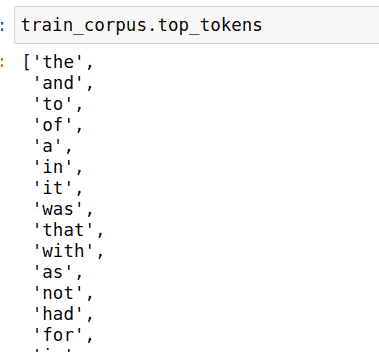Preview of the 50 tokens used for our stylometric analysis. Note that these are all function words, excluding pronouns (which could obviously vary between first- annd third-person writing styles and not be indicative of authorship).

## Calibrate the model and calculate the probability of each candidate in the training set being the author

Now the Burrows’ delta statistic above can be a little hard to interpret, ,and sometimes what we would like would be a probability value. How likely is Jane Austen to be the author of Sense and Sensibility?

We can do this by calibrating the model. The model looks at the Burrows’ delta values between known authors, works out what are the commonest values indicating same authorship:

`calibrate(train_corpus)`

After calling the calibrate method, we can now ask the model to give us the probabilities corresponding to the delta values in the above table:

`predict_proba(train_corpus, test_corpus)`

You can see that we now have a 76% probability that Villette was written by Charlotte Brontë.

As an aside: by default the library uses Scikit Learn’s Logistic Regression to calculate the calibration curve of the model. Alternatively, we could tell it which model to use by supplying an argument to the calibrate method:

## Plot the calibration curve

We can plot the calibration curve for a range of delta values:

```import numpy as np
import matplotlib.pyplot as plt
x_values = np.arange(0, 3, 0.1)```
```plt.plot(x_values, train_corpus.probability_model.predict_proba(np.reshape(x_values, (-1, 1)))[:,1])
plt.xlabel("Burrows delta")
plt.ylabel("Probability of same author")
plt.title("Calibration curve of the Burrows Delta probability model\nUsing Logistic Regression with correction for class imbalance")
```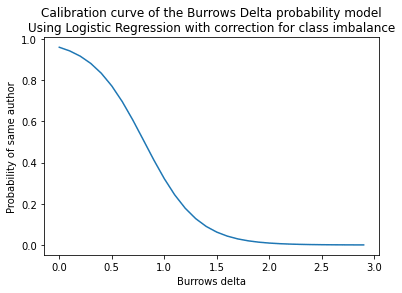Calibration curve of the Burrows Delta probability model, using Logistic Regression with correction for class imbalance

We can see that a value of 0 for delta would correspond to a near certainty that two books are by the same author, while a value of 2 corresponds to a near certainty that they are by different authors.

## Plot the ROC curve

The ROC curve and the AUC metric are useful ways of measuring the performance of a classifier.

The Burrows’ Delta method, when used as a two-class text classifier (different author vs. same author), has an incredibly easy task, because it has learnt from entire books. So we would expect the classifier to perform very well.

We can perform the ROC evaluation using cross-validation. The calibration code above has taken every book out of the training corpus in turn, trained a Burrows model on the remainder, and tested it against the withheld book. We take the probability scores resulting from this, and calculate the ROC curve.

An AUC score of 0.5 means that a classifier is performing badly, and 1.0 is a perfect score. Let’s see how well our model performs.

First, let’s get the ground truths (False = different author, True = same author) and Burrows’ delta values for all the comparisons that can be made within the training corpus:

`ground_truths, deltas = get_calibration_curve(train_corpus)`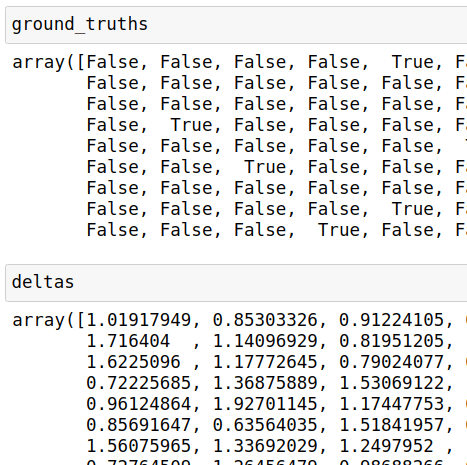The ground truths are Boolean values and the deltas are typically between 0 and 3. These delta values cannot be used directly to compute a ROC curve, since for that we would need probabilities.

We get the probabilities of each comparison the model has made by putting the Burrows’ delta values back through the trained Scikit-Learn model:

`probabilities = train_corpus.probability_model.predict_proba(np.reshape(deltas, (-1, 1)))[:,1]`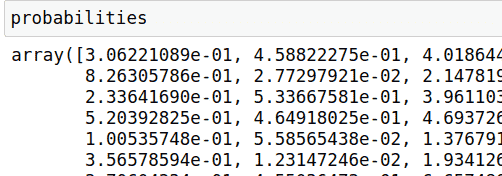The Scikit-Learn classifier has transformed the delta values into convenient probability values, lying between 0 and 1, which can be used to calculate the ROC curve.

We can put the probabilities and ground truths into Scikit-Learn to calculate the ROC curve:

```from sklearn.metrics import roc_curve, auc
fpr, tpr, thresholds = roc_curve(ground_truths, probabilities)```

We can now calculate the AUC score. If our model is good at identifying authorship, we should see a number close to 1.0.

`roc_auc = auc(fpr, tpr)`

Finally, we can plot the ROC curve:

```plt.figure()
plt.plot(fpr, tpr, color='darkorange',
lw=lw, label='ROC curve (area = %0.4f)' % roc_auc)
plt.plot([0, 1], [0, 1], color='navy', lw=2, linestyle='--')
plt.xlim([0.0, 1.0])
plt.ylim([0.0, 1.05])
plt.xlabel('False Positive Rate')
plt.ylabel('True Positive Rate')
plt.title('Receiver operating curve of the Burrows\' Delta classifier\noperating on entire books')
plt.legend(loc="lower right")
plt.show()```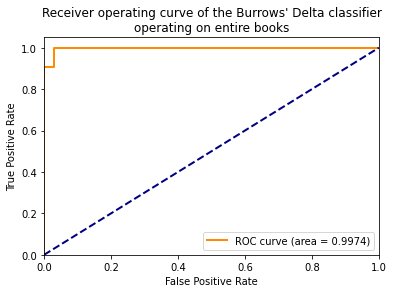The ROC curve of the Burrows’ delta classifier trained with cross-validation within the training set. Note the surprisingly high AUC, 0.9974, indicating that on this training set (entire books), we have something close to a perfect classifier.

## Segment the corpus and display the various books in a scatter graph using Principal Component Analysis

We can also visualise the stylistic similarities between the books in the training corpus, by calculating their differences and using Principal Component Analysis (PCA) to display them in 2D space.

For this, we need to use the Python machine learning library Scikit-Learn.

```from sklearn.decomposition import PCA
import re
import pandas as pd```

We can re-load the training corpus, and take segments of 80,000 words, so that we can include different sections of each book in our analysis.

```# Reload the training corpus as the "test corpus", re-tokenise it, and segment it this time
test_corpus = load_corpus_from_folder("faststylometry/data/train")
test_corpus.tokenise(tokenise_remove_pronouns_en)```
```split_test_corpus = test_corpus.split(80000)
```

Now we calculate the Burrows’ delta statistic on the book segments:

```df_delta = calculate_burrows_delta(train_corpus, split_test_corpus)
```

We are interested in the array of z-scores

`df_z_scores = split_test_corpus.df_author_z_scores`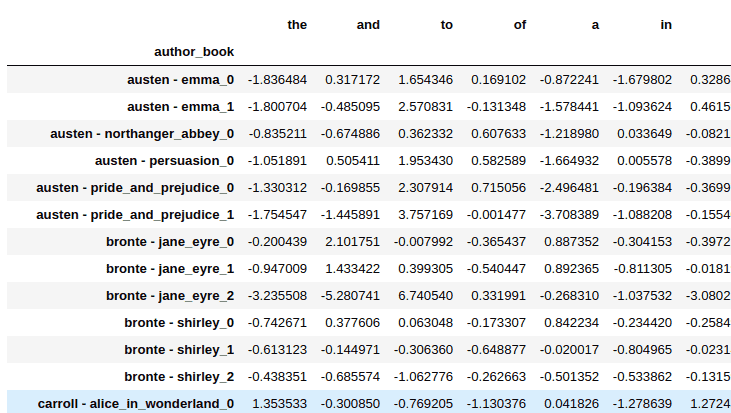The Burrows’ delta is calculated from z-scores of every common word in the vocabulary, which represent the fingerprint of the book and can be compared across books.

The above array is too big to display directly, so we need to reduce it to two-dimensional space to show it in a graph. We can do this using Scikit-Learn’s principal component analysis model, setting it to 2 dimensions:

`pca_model = PCA(n_components=2)`
`pca_matrix = pca_model.fit_transform(df_z_scores)`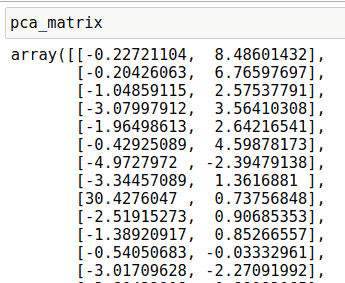Matrix of PCA coordinates for the books in our split test set, derived using PCA from the 50-dimensional Z-scores that were calculated for each book.

It would be nice to plot the book sections on a graph, using the same colour for every book by the same author. Since the Z-scores matrix is indexed by author and book name, we can use a regex to take everything before the first hyphen. This gives us the plain author name with the book title removed:

`authors = df_z_scores.index.map(lambda x : re.sub(" - .+", "", x))`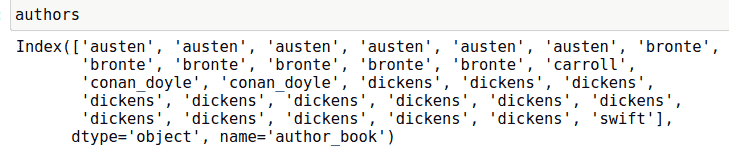The list of the authors’ names for all the book segments for which we have calculated the

We can join the PCA-derived coordinates and the author names together into one dataframe:

```df_pca_by_author = pd.DataFrame(pca_matrix)
df_pca_by_author["author"] = authors```

Now we can plot the individual books on a single graph:

```plt.figure(figsize=(15,15))

for author, pca_coordinates in df_pca_by_author.groupby("author"):
plt.scatter(*zip(*pca_coordinates.drop("author", axis=1).to_numpy()), label=author)
for i in range(len(pca_matrix)):
plt.text(pca_matrix[i], pca_matrix[i],"  " + df_z_scores.index[i], alpha=0.5)

plt.legend()

plt.title("Representation using PCA of works in training corpus")```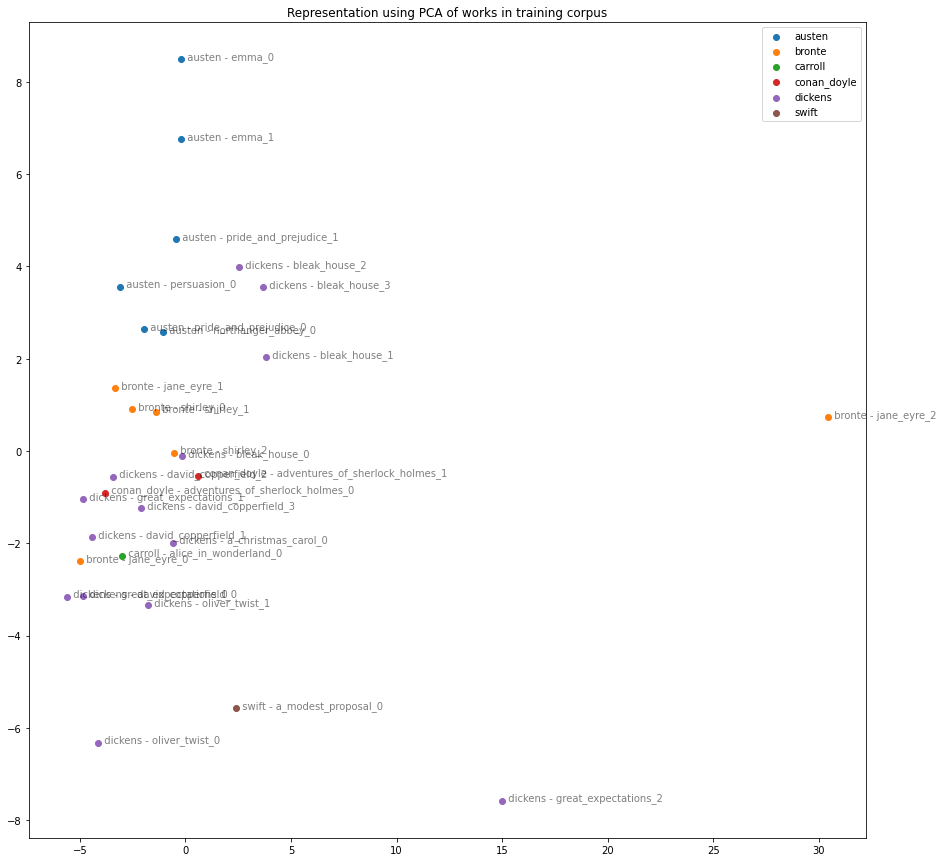Graph of the books in the training corpus, segmented into 80,000 tokens, with Burrows’ delta Z-scores calculated for the top 50 words, and reduced to 2 dimensions using PCA.

## Taking it further

The code for the ROC/AUC means that we can try out different parameters, changing the vocabulary size, document length, or preprocessing steps, to see how this affects the performance of the Burrows’ delta method.

I would be interested to find out how the delta performs on other languages, and if it would be beneficial to perform morphological analysis as a preprocessing step in the case of inflected languages. For example, in the Turkish word “teyzemle” (with my aunt), should this be treated as teyze+m+le, with the two suffixes input separately?

You can try this kind of experiment by replacing the function tokenise_remove_pronouns_en with a tokenisation function of your choice. The only constraint is that it must convert a string to a list of strings.